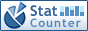Home PC Games Linux Windows Database Network Programming Server Mobile
 Home \ Programming \ The callback function used in C ++ - Memcached source installation and configuration under CentOS 6.6 (Server) - Vim useful plugin: vundle (Linux) - Linux Command-line MySQL summary (Database) - Android LayoutInflater source parsing (Programming) - Linux RPM (Linux) - To configure parameter configuration and software installation and uninstallation under Linux (Linux) - Ubuntu install Scala 2.10.x version (Linux) - Linux Log File Browser --logrotate (Linux) - Teach you how to protect the security of Linux desktop (Linux) - Unetbootin make use U disk loading Linux system (Linux) - Sublime Text 3 using summary (Linux) - Android first line of code study notes (Programming) - Linux 10 useful examples of command-line completion (Linux) - Docker + Nginx + Tomcat7 simple load balancing configuration (Server) - Oracle LONG RAW BLOB CLOB type of presentation (Database) - Dialogue UNIX:! \$ # @ *% (Linux) - Add your own kernel and ramfs based on an existing Linux LiveCD (Linux) - Java inheritance initialization problem (Programming) - Performance comparison Fibonacci recursive and non-recursive (Programming) - Specifies the open ports of the SUSE firewall settings (Linux) The callback function used in C ++ Add Date : 2016-06-06 Callback is a function call through a function pointer. If pointer A function as a parameter passed to B and then call the function A by A function passed in the function pointer in B, then that is a callback mechanism. A function is a callback function, and usually, A function is in your system meets the conditions set automatically. The callback function can use to improve the structure of the software, providing software reusability. Function pointer is a pointer, this pointer just like a normal pointer to a variable, then it points to a function, that is, it stores a pointer to a function. C ++ class member functions can not be used as a callback function as normal, because each member functions need to have an object instance to call it. Typically, to achieve a member function as a callback function, a common approach is to design the member function is a static member function. #include < iostream> typedef int (* Fun11) (int, int); typedef float (* Fun12) (float, float); int min (int a, int b) {  return a < b a:? b ;; } float max (float a, float b) {  return a> b a: b;? } int test1 () {  Fun11 pFun1 = NULL;  pFun1 = & min;  int ret1 = pFun1 (-1, 2);  std :: cout << "min value is:" << ret1 << std :: endl;  Fun12 pFun2 = NULL;  pFun2 = & max;  float ret2 = pFun2 (3.4, -2.2);  std :: cout << "max value is:" << ret2 << std :: endl;  return 0; } typedef void (* Fun2) (void *); class CallBack; class CallBackTest; class CallBackTest { public:  CallBackTest () {}  ~ CallBackTest () {}  void registerProc (Fun2 fptr, void * arg = NULL)  {   m_fptr = fptr;   if (arg! = NULL) {    m_arg = arg;   }  }  void doCallBack ()  {   m_fptr (m_arg);  } private:  Fun2 m_fptr;  void * m_arg; }; class CallBack { public:  CallBack (CallBackTest * t): a (2)  {   if (t) {    t-> registerProc ((Fun2) display, this);   }  }  ~ CallBack () {}  static void display (void * _this = NULL)  {   if (! _this) {    return;   }   CallBack * pc = (CallBack *) _ this;   pc-> a ++;   std :: cout << "a is" << pc-> a << std :: endl;  } private:  int a; }; int test2 () {  CallBackTest * cbt = new CallBackTest ();  CallBack * cb = new CallBack (cbt);  cbt-> doCallBack ();  return 0; } void callback31 () {  std :: cout << "this a callback function 31" << std :: endl; } int callback32 (int num) {  std :: cout << "this input param value is:" << num << std :: endl;  return 0; } void Caller31 (void (* ptr) ()) {  (* Ptr) (); } void Caller32 (int n, int (* ptr) (int)) {  (* Ptr) (n); } int test3 () {  Caller31 (callback31);  Caller32 (32, callback32);  return 0; } int main () {  test1 ();  test2 ();  test3 ();    return 0; } More:
 CopyRight 2002-2022 newfreesoft.com, All Rights Reserved.